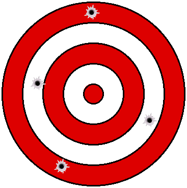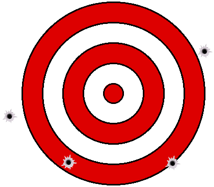## 1. Causes

A mistake is usually the result of carelessness or misunderstanding. Mistakes are generally isolated and stick out in a measurement set. These are common for people learning to use equipment or procedures for the first time - something gets forgotten, a wrong button is pushed, digits are transposed, etc.

## 2. Identifying and Compensating

The only way to know if a mistake exists is to repeat the measurement. Having just two measurements which differ greatly only indicates that there is a mistake, it doesn’t tell us which measurement has the mistake. To find out, we need at least a third measurement. Hopefully the third one will be close to one of the first two. If it is, we’ve found our culprit and would toss the wrong measurement. If it’s not, then we have a dilemma on our hands - evidently we’re doing something wrong, Figure D-1.a. Accurate, not precise b. Not accurate, not precise Figure D-1

Rather than count on luck, we use measurement procedures which allow us to identify mistakes. Repeating a measurement is part of the procedure but so is a precision. How much can measurements vary before we can identify ones with mistakes?

Assume we measure a tabletop length multiple times with these results (in inches): 48.5, 48.6, 58.0, 46.2, 48.2. The 59.0 measurement is considerably larger than the others; we can probably discard that one as containing a mistake. Do we accept the other four? The 46.2 measurement differs from the other three, but does it differ sufficiently? We need a precision to compare it against.

A common way to define precision in surveying is as a 1/X dimensionless ratio. The ratio is 1 unit variation across a magnitude of X. For example, 1/1000 means 1 ft variation in 1000 ft of measurement. Because it is dimensionless, it could also mean 1 meter per 1000 meters, 1 mile  per 1000 miles, etc. 1/1000 is better than 1/500; both have a 1 unit variation but the former occurs over 1000 while the latter 500.

A 1/X precision is a handy way to quickly evaluate a measurement set, particularly one with only a few measurements in it (we'll discuss statistical analysis a bit later). We can define a precision and any measurement which doesn't fall within it could be considered a mistake. We need to identify and eliminate mistakes because if not the mistakes are spread into all the measurements degrading the good ones.

Back to the tabletop example: let's use a precision of 1/100. The maximum variation of the remaining four measurements is 2.4, the average is 47.9. The precision of the measurement set is 2.4/47.9. Converted it to a 1/X ratio it is 1/20 which is much worse than 1/100. If we reject 4.62, we have a variation of 0.4 across an average of 48.4 for a precision of 1/121. It meets the precision and contains at least three measurements.

A precision specification must make sense in the context of how the measurements are made and the instrumentation used.